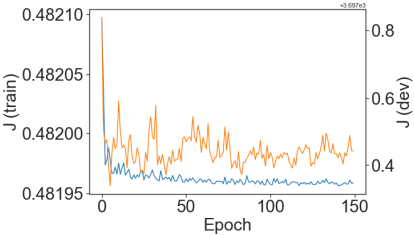# Network predicts the same curve independent of data-sample, why?

I define my Dataset class:

``````class MyDataset(Dataset):
def __init__(self, images, n, labels=None, transforms=None):
self.X = images
self.y = labels
self.n = n
self.transforms = transforms

def __len__(self):
return (len(self.X))

def __getitem__(self, i):
data = self.X.iloc[i, :]
#         print(data.shape)
data = np.asarray(data).astype(np.float).reshape(1,n,n)

if self.transforms:
data = self.transforms(data).reshape(1,n,n)

if self.y is not None:
y = self.y.iloc[i,:]
#             y = np.asarray(y).astype(np.float).reshape(2*n+1,) # for 257-vector of labels
y = np.asarray(y).astype(np.float).reshape(128,) # for 128-vector of labels
return (data, y)
else:
return data
``````

Then I create the instances of the train, dev, and test data:

``````train_data = MyDataset(train_images, n, train_labels, None)
dev_data = MyDataset(dev_images, n, dev_labels, None)
test_data = MyDataset(test_images, n, test_labels, None)
``````

After having trained my network, I want to see how well it behaves with the `test_data`. This is what I do to check this.

``````network = Network(128).double()

btch_sz = 100 #  batch size (e.g. 100)

images_test, labels_test = data
labels_test = labels_test.detach().numpy()
# to get the data samples from the first batch I break the for-loop cycle
break

outputs_test = network(images_test)
outputs_test = outputs_test.detach().numpy()
``````

Then I plot comparative graphs comparing predicted `outputs_test` with ground-truth `labels_test`:

``````nnn = 3 # to chose a specific sample from the first batch of the test dataset (e.g. 3rd data sample in the batch of 100 samples)

figure, ax1 = plt.subplots()
ax1.plot(labels_test[nnn-1,:], color=u'#1f77b4')
ax1.plot(160*outputs_test[nnn-1,:], color=u'#ff7f0e'')
ax1.legend(['True','Predicted'])
ax1.set_ylim([-40,40])
``````

As you can see, I have to multiply the `outputs_test` by 160 to make the resulting curve comparable in amplitude with the ground-truth curve. This is the first question, why would it be so?

Here are the plots for different data-samples from the batch of 100 and for different epochs of the training (the rows represents indicated epochs and the columns represent indicated data-samples from the batch):

As you can see, the result doesnt depend much on what data-sample is fed into the network. This is another question, why is that so?

Addon: For the reference this is how cost evolved over 150 epochs.Also, my network consists of 10 convolutional layers (including batchnorms and maxpools in some places) and 5 linear fully-connected layers.

This is the output of the `print(network)`:

``````Network(
(encoder): MyEncoder(
(conv_blocks): Sequential(
(0): Sequential(
(0): Conv2d(1, 6, kernel_size=(5, 5), stride=(1, 1))
(1): ReLU()
)
(1): Sequential(
(0): Conv2d(6, 12, kernel_size=(5, 5), stride=(1, 1))
(1): MaxPool2d(kernel_size=2, stride=2, padding=0, dilation=1, ceil_mode=False)
(2): BatchNorm2d(12, eps=1e-05, momentum=0.1, affine=True, track_running_stats=True)
(3): ReLU()
)
(2): Sequential(
(0): Conv2d(12, 32, kernel_size=(5, 5), stride=(1, 1))
(1): ReLU()
)
(3): Sequential(
(0): Conv2d(32, 32, kernel_size=(5, 5), stride=(1, 1))
(1): ReLU()
)
(4): Sequential(
(0): Conv2d(32, 16, kernel_size=(5, 5), stride=(1, 1))
(1): MaxPool2d(kernel_size=2, stride=2, padding=0, dilation=1, ceil_mode=False)
(2): BatchNorm2d(16, eps=1e-05, momentum=0.1, affine=True, track_running_stats=True)
(3): ReLU()
)
(5): Sequential(
(0): Conv2d(16, 16, kernel_size=(5, 5), stride=(1, 1))
(1): ReLU()
)
(6): Sequential(
(0): Conv2d(16, 14, kernel_size=(5, 5), stride=(1, 1))
(1): ReLU()
)
(7): Sequential(
(0): Conv2d(14, 14, kernel_size=(5, 5), stride=(1, 1))
(1): BatchNorm2d(14, eps=1e-05, momentum=0.1, affine=True, track_running_stats=True)
(2): ReLU()
)
(8): Sequential(
(0): Conv2d(14, 12, kernel_size=(5, 5), stride=(1, 1))
(1): ReLU()
)
(9): Sequential(
(0): Conv2d(12, 12, kernel_size=(5, 5), stride=(1, 1))
(1): MaxPool2d(kernel_size=2, stride=2, padding=0, dilation=1, ceil_mode=False)
(2): BatchNorm2d(12, eps=1e-05, momentum=0.1, affine=True, track_running_stats=True)
(3): ReLU()
)
)
)
(decoder): MyDecoder(
(fc_blocks): Sequential(
(0): Sequential(
(0): Linear(in_features=48, out_features=120, bias=True)
(1): ReLU()
)
(1): Sequential(
(0): Linear(in_features=120, out_features=60, bias=True)
(1): ReLU()
)
(2): Sequential(
(0): Linear(in_features=60, out_features=40, bias=True)
(1): BatchNorm1d(40, eps=1e-05, momentum=0.1, affine=True, track_running_stats=True)
(2): ReLU()
)
(3): Sequential(
(0): Linear(in_features=40, out_features=20, bias=True)
(1): ReLU()
)
(4): Sequential(
(0): Linear(in_features=20, out_features=10, bias=True)
(1): ReLU()
)
)
)
(last): Linear(in_features=10, out_features=128, bias=True)
)
``````

Your model doesn’t seem to learn the underlying distribution properly.
Try to scale down the problem by using a tiny dataset (e.g. just 10 sample) and make sure your model is able to overfit it by playing around with the architecture and hyperparameters.
Once this is done, you could scale up the use case again.

1 Like

Thanks a lot for this suggestion, @ptrblck! I will try it!

@ptrblck I tried your suggestion and I could achieve some sensitivity to the input data (I used the batch size of 1 and 1000 epochs). Also, the training data was fitted better than the test data, so it looks like overfitting. Although, the fitting of the training data is also not perfect as can be seen in the picture below.

And here is the prediction result for the two test data samples that my network has never seen before:I also tried the batch size of 10, and after 1000 epochs, the result is worse for the training data (compared to the batch size of 1):

For the same network architecture I tried the same images, but different “labels” (batch size of 1, 1000 epochs). The labels are oscillatory curves centered around zero vertically and horizontally. The result is not good at all: the prediction is the same for all 10 training data.

Question: Would you have an idea why oscillatory behaviour is so different?